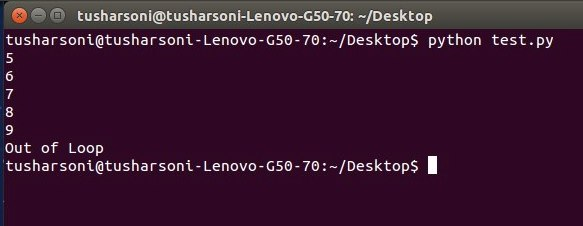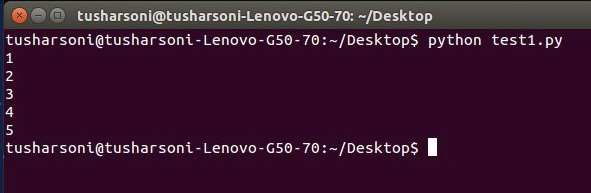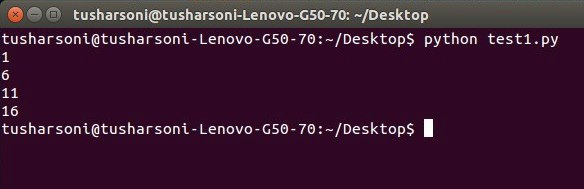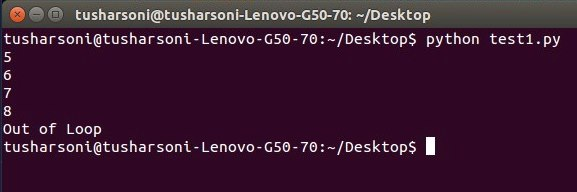# Python for & while Loop, break & continue Statement

In this tutorial you will learn about Python for & while loop, break & continue statement.

Loops are a fundamental part for any computer program if decisions are to be made. Python provides us an excellent and easy to use looping constructs. Lets look at the following looping structures in Python.

## Python while Loop

A while loop is used to execute a condition so long it gets evaluated to be true. It has following syntax.

Syntax

Example

OutputWe have initialized a variable ‘a’ to 5. Then we take a while construct and check for the condition if a is less than 10. For the first iteration, it is true and hence the statements within the loop executes and then value of a is incremented. On checking after the fifth iteration, the condition will be evaluated to false and control will come out of the loop. Hence, the print(“Out of Loop”) is executed.

Note that a=a+1 cannot be replaced by a++ in Python. You can also use a sentry variable in order to avoid the loop going into infinite mode. You need not use any braces or brackets in Python to define the loop structure. Proper indentations are prefect for Python interpreter to ascertain the scope of a loop.

## Python for Loop

The for loop is used for repetition of a particular lines of codes in a program. Suppose you want to print numbers until 10, you can do it either by typing 10 print statements or by using a for loop. The for loop repeats a part of a program based on the sequence.

for loop repeats its loop body for each of its elements in the given sequence. As soon as all the elements are executed, the loop terminates and the control comes out of its block.

Syntax

Example

OutputHere, a variable ‘a’ is defined with 5 values. The for loop here doesn’t check any condition as in case of a while loop. It just follows the sequence of a variable. We have also declared a counter variable ‘i’ which iterates throughout the loop. ‘in’ is a keyword used to mention the interpreter to loop through the variable ‘a’. Colon is necessary to tell the Python interpreter that the for loop block starts from next line onwards.

Note: Indentations are necessary as it helps the interpreter to identify the body of the for loop.

## Python range() Function

There is also another method or a function that can be used with for loop. Python provides a pre-defined library function named range(). It automatically creates a sequence on its definition in a Python program. It provides us the facility to execute statements after some pre-defined iterations or skips. You can understand from the following example.

Example

OutputHere, range() is used to create a sequence starting from 1 and it ends at 20. But each time the value of counter variable ‘i’ will be increased by 5.

## Python break and continue Statement

break and continue statements can be used in while and for loops. break statement terminates the loop execution and the control immediately come out of loop body. continue statement makes the loop to skip the remainder of its body and immediately retest its condition prior to reiterating.

Example

OutputThe above example demonstrates the use of a break statement in a while loop. Here, the value of a will be incremented until 8 and then the break statement will be executed and the control will come out of loop.

### 2 thoughts on “Python for & while Loop, break & continue Statement”

1.Hii Neeraj,

I am learning with the tutorial provided for Python. i am unable to understand the difference between range and in function used with for loop

2.Hi ritesh
‘in’ is used when we define a list first and then we want to loop through the items in the list.
a={1,2,3,4,5}
Here ‘a’ is a list and to loop through this we say
for i in a
print i
Here the variable ‘i’ will loop through ‘a’.So the first value of ‘i’ will be 1 and then 2…. till it reaches 5.

In case of range() function we are specifying the beginning and last value for it to loop through.So if we see the for loop example given in this blog by Neeraj
for i in range(1,20,5)
print i
Here the initial value of ‘i’ is 1 and the final value is 20.After the final value the number 5 here says that each time the loop executes it should increment by 5.
So first i will be 1, then 1+5=6,6+5=11 and so on.
If we do not specify by what value it should increment the value of ‘i’ increments by 1 which is the default.